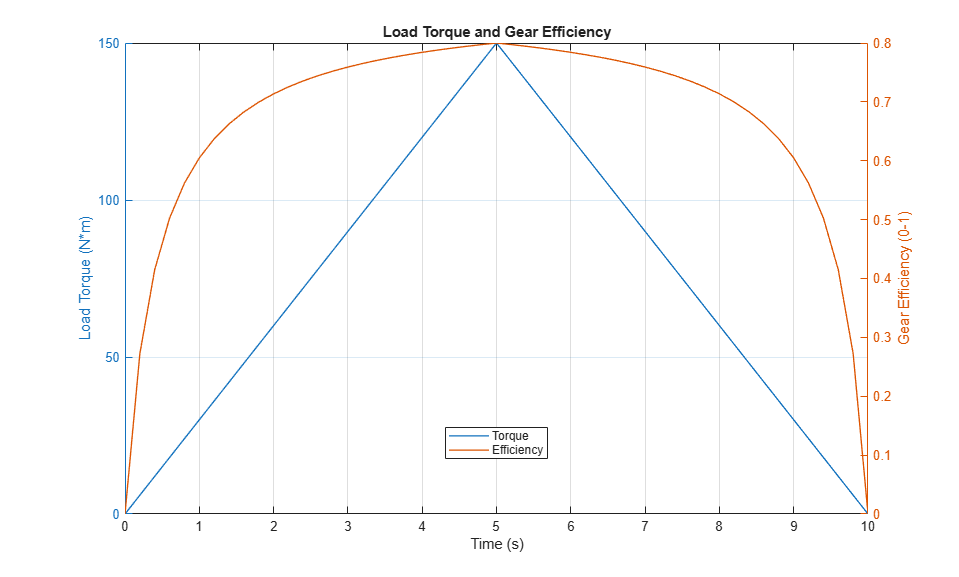# Gearbox Efficiency Measurement

This example shows a test for measuring gear box efficiency. The input shaft is driven at a fixed speed and a variable torque is applied to the output shaft. Power is measured on both the input and output shafts, and efficiency is calculated as the ratio of output to input power. The simulation results match the parameters specified for the efficiency of the gear.

### Model### Simulation Results from Simscape Logging

The plot below shows load-dependent efficiency in a gear model. A gear is driven at a constant speed as the load torque is varied. The efficiency at the nominal point exactly matches the parameter values in the block.